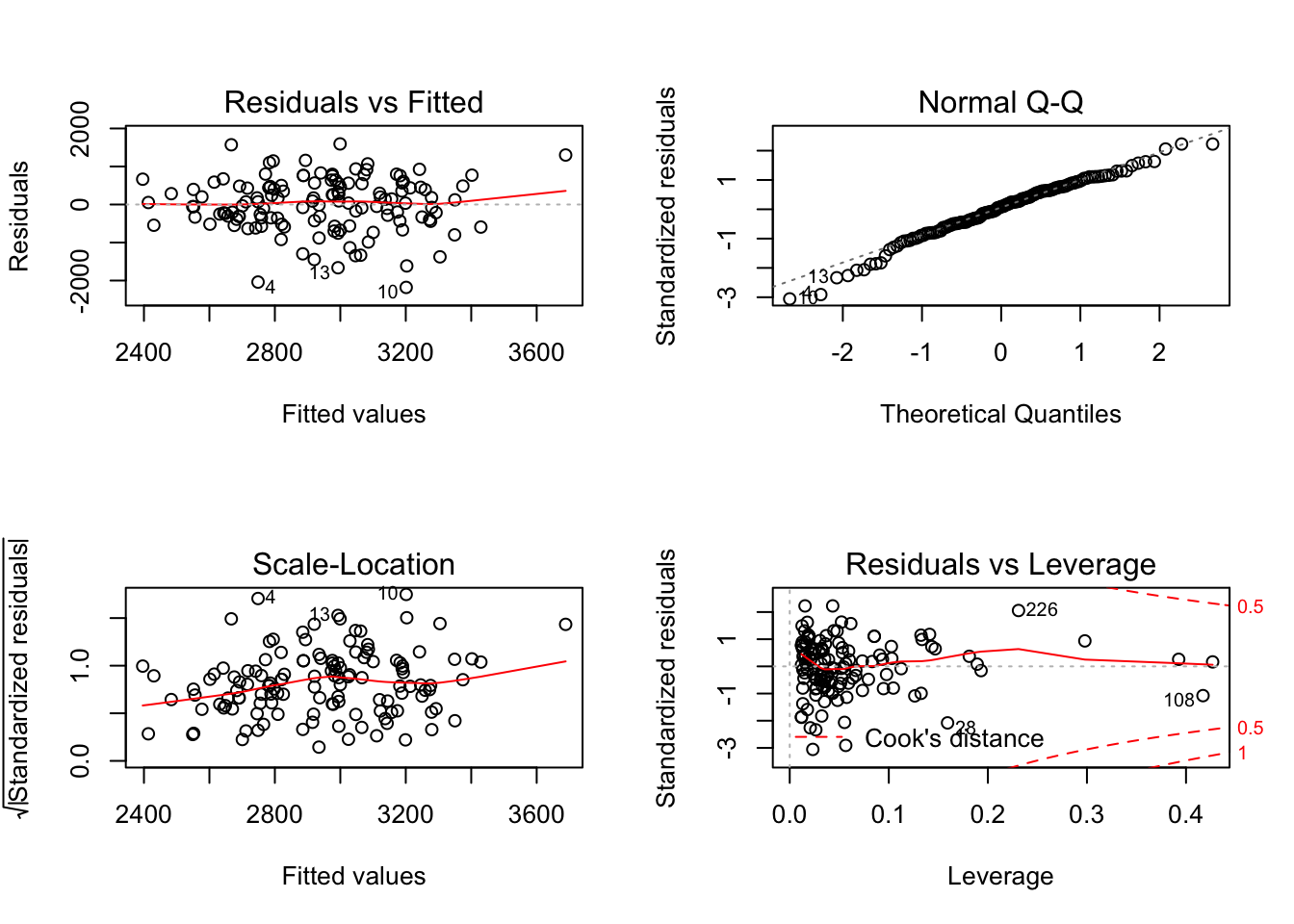# Packages

For this lab, use only methods availible from the following packges:

library(MASS)
library(tidyverse)
library(knitr)
library(kableExtra)

If you haven’t already, make sure each is installed!

# Data

For this lab we will use the birthwt data from the MASS package. Our goal in analyzing this data is to predict the birthweight of newborns at Baystate Medical Center, Springfield, Mass. (This data is from 1986…)

data(birthwt)

[Exercise] Read the documentation for this data. Based on our goal, one of the variables should not be used in our analysis. Remove this variable.

birthwt = subset(birthwt, select = -c(low))

[Exercise] Based on reading the documentation, it should be clear that some of the variables are categorical. Coerce these variables to be factor variables.

We will always encode categorical data as factors when using formula syntax to specify a model. For full details on factor variables, see the Factors chapter from Hadley Wickham’s book R for Data Science.

birthwt$race = factor(ifelse(birthwt$race == 1, "white",
ifelse(birthwt$race == 2, "black", "other"))) birthwt$smoke = factor(birthwt$smoke) birthwt$ht = factor(birthwt$ht) birthwt$ui = factor(birthwt$ui) [Exercise] After completing the previous preparations, test-train split the data using the following code: set.seed(42) bwt_trn_idx = sample(nrow(birthwt), size = trunc(0.70 * nrow(birthwt))) bwt_trn_data = birthwt[bwt_trn_idx, ] bwt_tst_data = birthwt[-bwt_trn_idx, ] # Model Training Let black, white, other, and smoke each be dummy variables. For example white is 1 is the mother’s race is white, otherwise it is 0. [Exercise] Fit (train) a total of five models: • An additive model using all availible predictors • A model using all availible predictors as well as all possible two-way interactions • $$\texttt{bwt} = \beta_0 + \beta_1 \texttt{age} + \beta_2 \texttt{lwt}+ \epsilon$$ • $$\texttt{bwt} = \beta_0 + \beta_1 \texttt{age} + \beta_2 \texttt{lwt} + \beta_3 \texttt{other} + \beta_3 \texttt{white} + \epsilon$$ • $$\texttt{bwt} = \beta_0 + \beta_1 \texttt{age} + \beta_2 \texttt{lwt} + \beta_3 \texttt{smoke} \\ + \beta_4 (\texttt{age}\times\texttt{lwt}) + \beta_5 (\texttt{age}\times\texttt{smoke}) + \beta_6 (\texttt{lwt}\times\texttt{smoke}) + \beta_7 (\texttt{age}\times\texttt{lwt}\times\texttt{smoke}) + \epsilon$$ bwt_mod_1 = lm(bwt ~ ., data = bwt_trn_data) bwt_mod_2 = lm(bwt ~ . ^ 2, data = bwt_trn_data) bwt_mod_3 = lm(bwt ~ age + lwt, data = bwt_trn_data) bwt_mod_4 = lm(bwt ~ age + lwt + race, data = bwt_trn_data) bwt_mod_5 = lm(bwt ~ age * lwt * smoke, data = bwt_trn_data) # Model Evaluation Consider two evaluation metrics, RMSE and $$R^2$$, which can both be evaluated on train or test data: $\text{RMSE}(\hat{f}, \mathcal{D}) = \sqrt{ \frac{1}{n_\mathcal{D}} \sum_{i \in \mathcal{D}} (y_i - \hat{f}(x_i))^2}$ $R^2(\hat{f}, \mathcal{D}) = 1 - \frac{\sum_{i \in \mathcal{D}} (y_i - \hat{f}(x_i))^2}{\sum_{i \in \mathcal{D}} (y_i - \bar{y})^2}$ In both cases, $$\hat{f}$$ is a model that has been fit to training data, thus $$\hat{f}(x_i)$$ is the model’s prediction given the predictors for observation $$i$$ from dataset $$\mathcal{D}$$, which could be either test or train. Similiarly $$y_i$$ is the true value of the response for observation $$i$$ from dataset $$\mathcal{D}$$ We supply R functions to calculate both. # RMSE calc_lm_rmse = function(mod, data) { actual = data$bwt
predicted = predict(mod, data)
sqrt(mean((actual - predicted) ^ 2))
}

# R2
calc_lm_r2 = function(mod, data) {
actual = data\$bwt
predicted = predict(mod, data)
1 - ((sum((actual - predicted) ^ 2)) / (sum((actual - mean(actual)) ^ 2)))
}

Note that these functions are specific both to the models we are using and the data we are using. They should not be re-used outside of this lab! Don’t just copy and paste them into homework and expect them to do anything useful!

[Exercise] Calculate test and train RMSE for each model.

# create model list
mod_list = list(bwt_mod_1, bwt_mod_2, bwt_mod_3, bwt_mod_4, bwt_mod_5)
trn_rmse = sapply(mod_list, calc_lm_rmse, data = bwt_trn_data)
tst_rmse = sapply(mod_list, calc_lm_rmse, data = bwt_tst_data)

Here we used the sapply() function. A couple useful resources for learning the *apply() functions:

[Exercise] Calculate test and train $$R^2$$ for each model.

# create model list
trn_r2 = sapply(mod_list, calc_lm_r2, data = bwt_trn_data)
tst_r2 = sapply(mod_list, calc_lm_r2, data = bwt_tst_data)

[Exercise] Summarize these results in a table. (Model, Train/Test RMSE, Train/Test $$R^2$$.) Output the results as a well-formatted markdown table.

# create df of results
results = data.frame(
mod = c("bwt ~ .", "bwt ~ . ^ 2", "bwt ~ age + lwt",
"bwt ~ age + lwt + race", "bwt ~ age * lwt * smoke"),
trn_rmse = trn_rmse,
tst_rmse = tst_rmse,
trn_r2 = trn_r2,
tst_r2 = tst_r2
)
colnames(results) = c("Model", "Train RMSE", "Test RMSE", "Train R2", "Test R2")

# create results table
kable_styling(kable(results, format = "html", digits = 3), full_width = FALSE)
Model Train RMSE Test RMSE Train R2 Test R2
bwt ~ . 610.066 700.586 0.320 -0.008
bwt ~ . ^ 2 509.609 1223.517 0.525 -2.076
bwt ~ age + lwt 716.231 722.785 0.062 -0.073
bwt ~ age + lwt + race 687.964 731.563 0.135 -0.100
bwt ~ age * lwt * smoke 699.546 684.335 0.106 0.038

# Results?

[Exercise] You might have noticed that you would select the same model using RMSE or $$R^2$$. So $$R^2$$ is as good as RMSE, right?

No!!!* $$R^2$$ is in some arbitrary units, whereas RMSE gives us an estimate of the average squared prediction error in the original units used by the response variable!

[Exercise] Create a 95% prediction interval for the first observation in the test dataset using the best model of these five. Do you feel that this interval is valid?

predict(bwt_mod_5, bwt_tst_data[1, ], interval = c("prediction"), level = 0.95)
##         fit      lwr      upr
## 87 2561.925 1097.957 4025.893
par(mfrow = c(2, 2))
plot(bwt_mod_5)The diagnostics look reasonable, so we don’t have any reason to doubt the interval’s validty. However, with such a large interval, we should question the usefulness of our predictions!